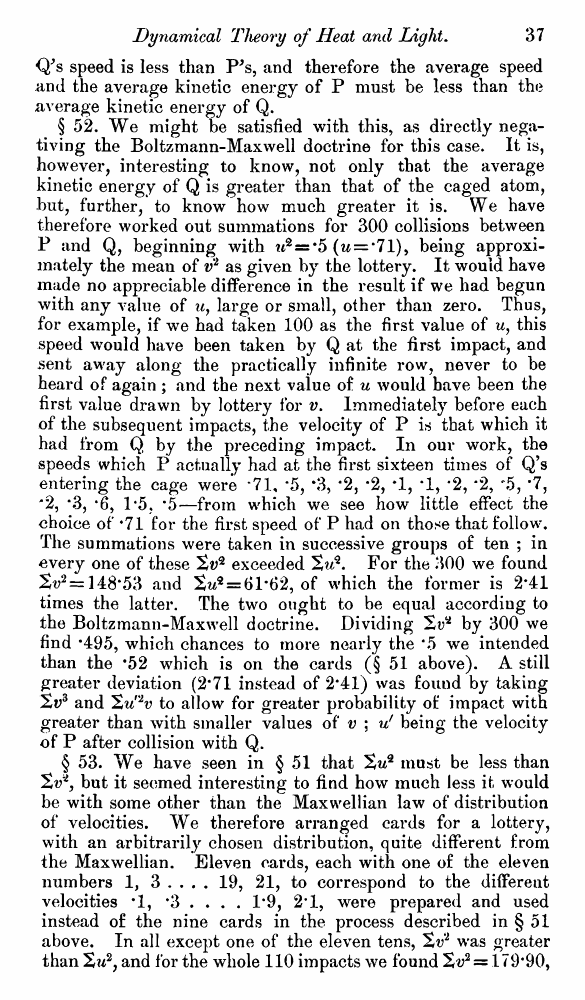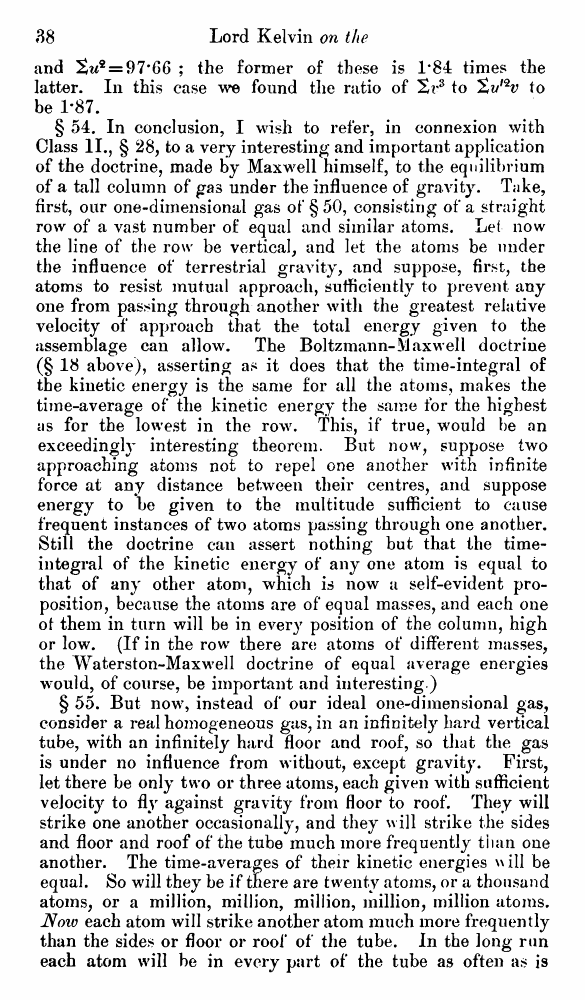# Lord Kelvin. Nineteenth-Century Clouds over the Dynamical Theory of Heat and Light. // Phil. Mag. S. 6. Vol. 2. No. 7. July 1901.

В начало   Другие форматы   <<<     Страница 37   >>>1  2  3  4  5  6  7  8  9  10  11  12  13  14  15  16  17  18  19  20  21  22  23  24  25  26  27  28  29  30  31  32  33  34  35  36  37 38  39  40 Q’s speed is less than P's, and therefore the average speed and the average kinetic energy of P must be less than the average kinetic energy of Q. § 52. We might be satisfied with this, as directly negativing the Boltzmann-Maxwell doctrine for this case. It is, however, interesting to know, not only that the average kinetic energy of Q is greater than that of the caged atom, but, further, to know how much greater it is. We have therefore worked out summations for 300 collisions between P and Q, beginning with w2=s*5 (w = *71), being approximately the mean of v2 as given by the lottery. It would have made no appreciable difference in the result if we had begun with any value of u, large or small, other than zero. Thus, for example, if we had taken 100 as the first value of u, this speed would have been taken by Q at the first impact, and sent away along the practically infinite row, never to be heard of again ; and the next value of u would have been the first value drawn by lottery for v. Immediately before each of the subsequent impacts, the velocity of P is that which it had from Q by the preceding impact. In our work, the speeds which P actually had at the first sixteen times of Q’s entering the cage were *71, *5, *3, *2, *2, *1, *1, *2, *2, “5, *7, '2, *3, *6, 1*5, *5—from which we see how little effect the choice of *71 for the first speed of P had on those that follow. The summations were taken in successive groups of ten ; in every one of these St;2 exceeded Xu*. For the 300 we found 2^*= 148*53 and 2w2 = 61#62, of which the former is 2*41 times the latter. The two ought to be equal according to the Boltzmann-Maxwell doctrine. Dividing by 300 we find *495, which chances to more nearly the *5 we intended than the *52 which is on the cards (§ 51 above). A still greater deviation (2*71 instead of 2*41) was found by taking 2v3 and 'Zu'2v to allow for greater probability of impact with greater than with smaller values of v ; v! being the velocity of P after collision with Q. § 53. We have seen in § 51 that %uq must be less than Sv*, but it seemed interesting to find how much less it would be with some other than the Maxwellian law of distribution of velocities. We therefore arranged cards for a lottery, with an arbitrarily chosen distribution, quite different from the Maxwellian. Eleven cards, each with one of the eleven numbers 1, 3 . . . . 19, 21, to correspond to the different velocities *1, *3 . . . . 1*9, 2*1, were prepared and used instead of the nine cards in the process described in § 51 above. In all except one of the eleven tens, was greater than %u2, and for the whole 110 impacts we found 2v2 = 179*90, and '2tU* = 97'66 ; the former of these is 1*84 times the latter. In this case we found the ratio of to ^Zv,<2v to be 1*87. § 54. In conclusion, I wish to refer, in connexion with Class II., § 28, to a very interesting and important application of the doctrine, made by Maxwell himself, to the equilibrium of a tall column of gas under the influence of gravity. Take, first, our one-dimensional gas of § 50, consisting of a straight row of a vast number of equal and similar atoms. Lei now the line of the row be vertical, and let the atoms be under the influence of terrestrial gravity, and suppose, first, the atoms to resist mutual approach, sufficiently to prevent any one from passing through another with the greatest relative velocity of approach that the total energy given to the assemblage can allow. The Boltzmann-Maxwell doctrine (§ 18 above), asserting as it does that the time-integral of the kinetic energy is the same for all the atoms, makes the time-average of the kinetic energy the same for the highest as for the lowest in the row. This, if true, would be an exceedingly interesting theorem. But now, suppose two approaching atoms not to repel one another with infinite force at any distance between their centres, and suppose energy to be given to the multitude sufficient to cause frequent instances of two atoms passing through one another. Still the doctrine can assert nothing but that the time-integral of the kinetic energy of any one atom is equal to that of any other atom, which is now a self-evident proposition, because the atoms are of equal masses, and each one of them in turn will be in every position of the column, high or low. (If in the row there are atoms of different masses, the Waterston-Maxwell doctrine of equal average energies would, of course, be important and interesting.) § 55. But now, instead of our ideal one-dimensional gas, consider a real homogeneous gas, in an infinitely hard vertical tube, with an infinitely hard floor and roof, so that the gas is under no influence from without, except gravity. Fii*st, let there be only two or three atoms, each given with sufficient velocity to fly against gravity from floor to roof. They will strike one another occasionally, and they will strike the sides and floor and roof of the tube much more frequently than one another. The time-averages of their kinetic energies w ill be equal. So will they be if there are twenty atoms, or a thousand atoms, or a million, million, million, million, million atoms. Noiv each atom will strike another atom much more frequently than the sides or floor or roof of the tube. In the long run each atom will be in every part of the tube as often as is